#### Subject 2. Macaulay, Modified and Effective Durations

Bond duration measures the sensitivity of the full price change to a change in interest rates.

• Yield duration statistics measure the sensitivity of a bond's full price to the bond's own yield-to-maturity. They include the Macaulay duration, modified duration, money duration, and price value of a basis point.
• Curve duration statistics measure the sensitivity of a bond's full price to the benchmark yield curve, e.g., effective duration.

Duration is the weighted average time to receive the present value of each of the bond's coupon and principal payments. For example, a bond with a duration of three means that, on average, it takes three years to receive the present value of the bond's cash flows.

Macaulay Duration

Frederick Macaulay developed the concept of duration approximately 80 years ago. He demonstrated that a bond's duration was a more appropriate measure of time characteristics than the term to maturity of the bond, because duration incorporates both the repayment of capital at maturity, the size of the coupon and timing of the payments.

Macaulay duration is defined as the weighted average time to full recovery of principal and interest payments. The weights are the shares of the full price corresponding to each coupon and principal payment.

Alternatively, Macaulay duration can be calculated using a closed-form formula.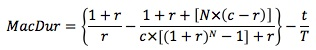Modified Duration

Modified duration shows how bond prices move proportionally with small changes in yields. Specifically, modified duration estimates the percentage change in bond price with a change in yield.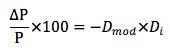-Dmod = the modified duration for the bond
Di = yield change in basis points divided by 100
P = beginning price for the bond

Modified duration assumes that the price/yield relationship is a straight line. However, the price/yield relationship is convex, not linear. Suppose that the bond has an initial yield of Y0. A tangent line can be drawn to the price/yield relationship at Y0. The slope of the tangent line is related to the duration of the bond. If the yield falls to Y1, the price will rise to P1. Due to the linear assumption, the price change measured by duration is P2 - P0.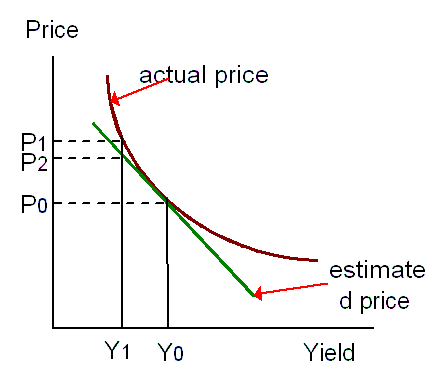To approximate modified duration: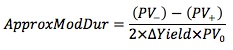V- = the price if yields declines
V+ = the price if yield increases
V0 = the initial price

For example, consider a 9% coupon 20-year option-free bond selling at 134.6722 to yield 6%. If the yield is decreased by 20 basis points from 6.0% to 5.8%, the price would increase to 137.5888. If the yield increases by 20 basis points, the price would decrease to 131.8439. Thus: ApproxModDur = (137.5888 - 131.8439)/(2 x 134.6722 x 0.002) = 10.66. This tells you that for a 1% change in the required yield, the bond price will change by approximately 10.66%.

Macaulay duration is mathematically related to modified duration.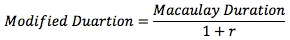A bond with a Macaulay duration of 10 years, a yield to maturity of 8% and semi-annual payments will have a modified duration of:
Dmod = 10/(1 + 0.08/2) = 9.62 years

Effective Duration

Effective duration measures interest rate risk in terms of a change in the benchmark yield curve. It is very similar to approximate modified duration.A pricing model can be used to estimate the price change resulting from a change in the benchmark yield curve instead of the bond's own yield-to-maturity. V- and V+ are adjusted to reflect any changes in the cash flows (due to embedded options) that result from the change in benchmark yield curve.

Effective duration should be used for bonds with embedded options.

Key-Rate Durations

It is important to distinguish interest rate risk from yield curve risk.

• The interest rate risk is the sensitivity of a bond to parallel shifts of the yield curve.
• The yield curve risk is a bond's sensitivity to changes in the shape of the yield curve.

Parallel shifts in the yield curve rarely occur. An analyst may want to measure the change in the bond's price by changing the spot rate for a particular key maturity and holding the spot rate for the other key maturities constant. The key rate duration is the sensitivity of the value of a bond to changes in a single spot rate, holding all other spot rates constant. There is a key rate duration for every point on the spot rate curve so there is a vector of durations representing each maturity on the spot rate curve.